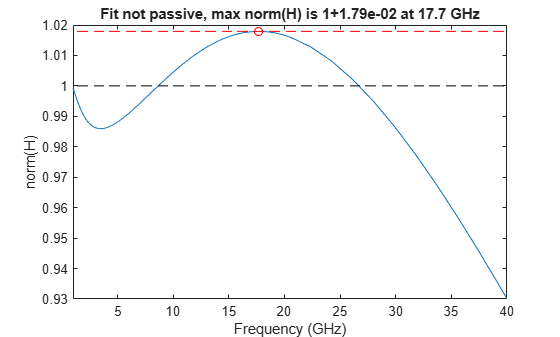# passivity

Plot passivity of N-by-N `rationalfit` function output

Since R2019a

## Syntax

``passivity(fit)``
``passivity(fit,xlimits)``
``[maxfreq,maxvalue,freqs,ns] = passivity(fit)``

## Description

example

````passivity(fit)` plots the passivity of the input, `fit`, over a range of frequencies. Passivity is measured by computing the H-infinity norm of the fit. H-infinity norm is the maximum two-norm of the transfer function `H` over all the frequencies (0, Inf).```
````passivity(fit,xlimits)` plots the passivity with X-axis limits of the plot.```
````[maxfreq,maxvalue,freqs,ns] = passivity(fit)` returns the data that is used to generate the plot.```

## Examples

collapse all

Read the file, `passive.s2p` and fit the 2-by-2 S-parameters.

```S = sparameters('passive.s2p'); fit = rationalfit(S);```

Test the passivity of the 2-by-2 fit.

`ispassive(fit)`
```ans = logical 0 ```

Plot the passivity of the 2-by-2 fit.

```figure passivity(fit,[1e9 40e9])```## Input Arguments

collapse all

Rational fit of S-parameters of passive elements, specified as an N-by-N array of `rfmodel.rational` objects as returned by the `rationalfit` function or a `rational` object with S-parameters as input.

Data Types: `double`

X-axis limits of the plot, specified as a 1-by-2 vector.

Data Types: `double`

## Output Arguments

collapse all

Maximum norm(H) over the frequencies (0, Inf), returned as a scalar.

Data Types: `double`

Frequency at which norm(H) is equal to maximum value, returned as a scalar in hertz.

Data Types: `double`

Two-norm of the transfer function H, returned as a column vector.

Data Types: `double`

Frequency values, returned as a column vector.

Data Types: `double`

## Version History

Introduced in R2019a